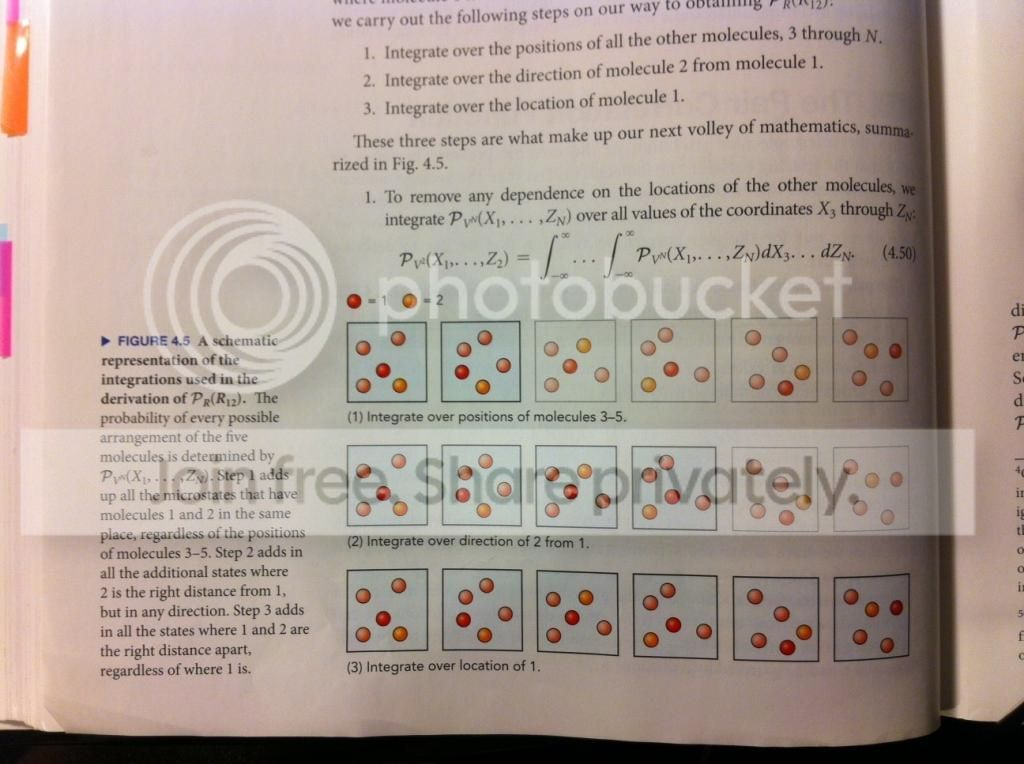# In statistical mechanics, what does integrating with respect to the position of a molecule mean?

So, this is probably a dumb question, but I cannot visualize or make sense of integrating over the position of a molecule in space. Okay, so an example in my thermodynamics textbook: we have N = 5 molecules and we want to find the probability of finding two specific molecules, 1 and 2, separated a distance R from each other. It says that we need to use the probability distribution function to get the probability per unit volume of locating a specific molecule 1 in our sample of N molecules. I took a picture of this page from my textbook, which I'm sorry about but I'm not too sure on how to properly include mathematical notations on this site:I guess what I really want to know is why does integrating over dX3...dZn eliminate dependence on the other molecules besides molecules 1 and 2? (Eq. 4.50) Thanks in advance.

Let me simplify the problem: assume a container of unit LENGTH along the X axis, assume that we have only 3 molecules instead of 5, and that the molecules move only along the X axis, i.e. they don't have a transversal velocity.

Now, what you need to find (in my understanding) is the probability to find the molecules 1 and 2 separated by a distance R.

Let's first calculate the probability to find the molecule 1 at a certain point q, and the molecule 2 at the point q+R. For this purpose let's divide the axis $\mathbf{X}$ inside the container into small intervals of equal length, located around the points $x_0$, $x_0 + d$, $x_0 + 2d$, etc. Let for example the point q be in the interval around the point $x_0 + 9d$, and the point $q+R$ in the interval $x_0 + 15d$ . Now the probability to have the molecules 1 and 2 in the above intervals respectively, is equal to:

Prob. to have $1$ and $2$ in the above intervals AND molecule $3$ in the interval around $x_0$ +

Prob. to have $1$ and $2$ in the above intervals AND molecule $3$ in the interval around $x_0+d$ +

Prob. to have $1$ and $2$ in the above intervals AND molecule $3$ in the interval around $x_0+2d$ +

Prob. to have $1$ and $2$ in the above intervals AND molecule $3$ in the interval around $x_0+3d$ etc...

Mathematically,

$P(q_{1} = x_{0}+9d, q_{2}=x_0 + 15d) = P(q_{1}-q_{2} = R, q_{3} = x_{0}) + P(q_{1}-q_{2} = R, q_{3} = x_{0}+d) + ..... P(q_{1}-q_{2} = R, q_{3} = x_{0}+15 d)$,

where $x_{i}, i = 1,2,3$ represents the position of the $i^{th}$ particle and $x_{0}$ represents and arbitrary origin with $d$ as the discretization in space.

When the length d of these intervals tends to zero, the above sum becomes an integral of the distribution probability, with the molecules 1 and 2 fixed at the above positions. The integral is over the position of the molecule 3.

Now, please generalize for having N molecules and a 3dimensional container. For calculating in continuation the probability of a distance R between the molecules 1 and 2, you follow the steps 2 and 3 on your photographed page.

I hope it helps,

Sofia